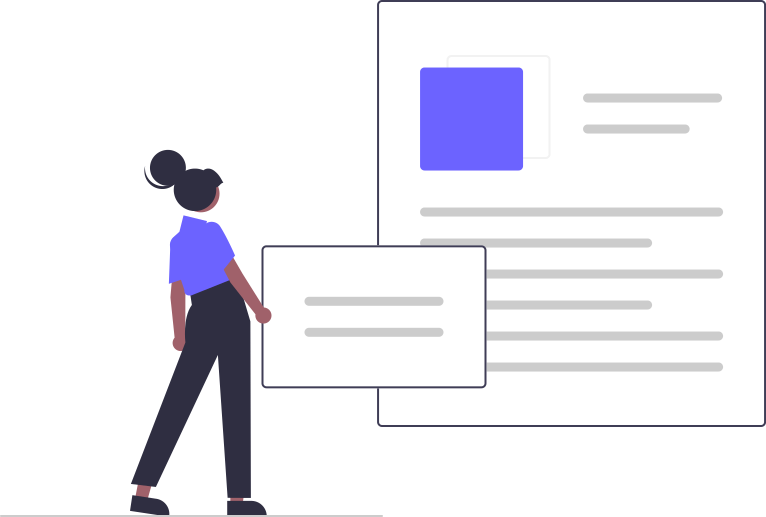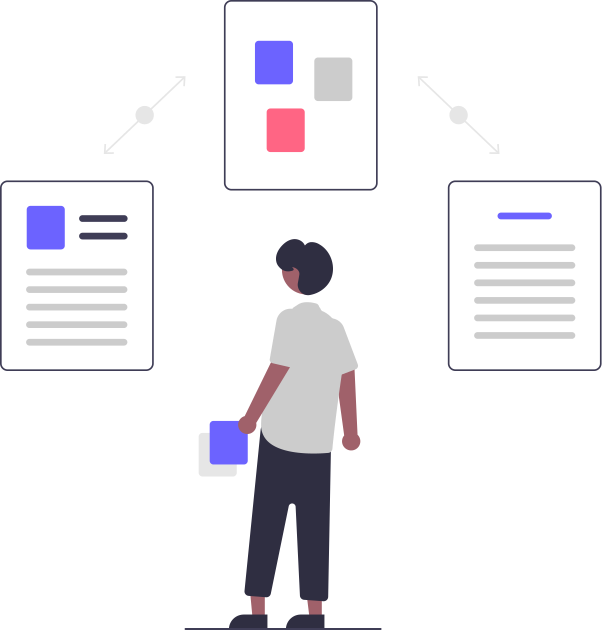# Text & Math OCR

Built for Science and Maths

Stop doing trial and error with LaTeX just scan the equation and get LaTeX, copy the output and paste wherever you need.

## Text & Math OCR

e_{i, j}=\left(\mathbf{h}_{i}^{x}\right)^{T} \cdot \mathbf{h}_{j}^{y}
\int \frac{x d x}{\sqrt{3 x^{2}-11 x+2}} .
\tan \left(\theta+\frac{\pi}{4}\right)=3 \tan 3 \theta
\left[\frac{x_{1}+x}{2}, \frac{y_{1}-y_{2}}{2}\right]s
\text { Find } x, y, z \text { if } A=\left[\begin{array}{ccc} 0 & 2 y & z \\ x & y & -z \\ x & -y & z \end{array}\right] \text { satisfies } A^{\prime}=A^{-1} .
\cos ^{2} \theta\left(1+\tan ^{2} \theta\right)=1

## Developer Friendly

 import axios from 'axios'; const payload = { src : 'data:image/png;base64,iVBO...', // You can provide other parameters also // like scaleX, scaleY. Check Documentation } const headers = { 'Authorization' : YOUR_TOKEN_HERE } axios.post('https://developer.trysolvio.ai/api/text/digital',payload, headers).then(data => { console.log(data) }) 
Well Documented APIs
We are also developers and so we know what problems developers face. We have great documentation with examples. If you face any diffculty we are here to help you

## Why Us?Trained on millions of Text and Math datapoints99.99% Uptime and Low LatencyNeed something extra? We provide custom deployments too!

## Use CasesContent Import